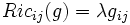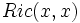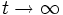# Einstein metric

(Redirected from Einstein manifold)

This article defines a property that makes sense for a Riemannian metric over a differential manifold

This property of a Riemannian metric is Ricci flow-preserved, that is, it is preserved under the forward Ricci flow

This is the property of the following curvature being constant: Ricci curvature

## Definition

### Symbol-free definition

A Riemannian metric on a differential manifold is said to be an Einstein metric if the Ricci curvature tensor is proportional to the metric tensor.

### Definition with symbols

Let$(M,g)$ be a Riemannian manifold.$g$ is teremd an Eisetin metric if it satisfies the following equivalent conditions:

•$Ric_{ij}(g) = \lambda g_{ij}$

where$\lambda$ is uniform for the whole manifold.

This value of$\lambda$ is termed the cosmological constant for the manifold.

•$Ric(x,x)$ is constant for all unit tangent vectors at all points. In other words, the Ricci curvature is constant for all one-dimensional subspaces.

This constant is the same$\lambda$ as above -- the cosmological constant.

### For pseudo-Riemannian manifolds

We can also talk of whether a pseudo-Riemannian metric is an Einstein metric. Further information: Einstein pseudo-Riemannian metric

## Relation with other properties

### Stronger properties

The following properties of Riemannian metrics are stronger than the property of being an Einstein metric:

• Ricci-flat metric: This is an Einstein metric with cosmological constant zero, that is, with Ricci curvature zero everywhere
• Constant-curvature metric: This is a metric with the property that the sectional curvature for all 2-dimensional subspaces being equal. The implication holds because the Ricci curvature associated with a direction is a sum of sectional curvatures of planes containing that direction, and all the sectional curvatures in turn are constant.

### In low dimensions

The following turn out to be true:

• For manifolds of dimension upto three, Einstein metrics are precisely the same as constant-curvature metrics

## Flows

### volume-normalized Ricci flow

The Riemannian metrics with this property are precisely the stationary points for this flow: volume-normalized Ricci flow

The volume-normalized Ricci flow is a flow on the space of all Riemannian metrics on a differential manifold, for which the stationary points are precisely the Einstein metrics.

The interest in Ricci flows in the context of Einstein metrics arises from the following general question: given a differential manifold, can we associate an Einstein metric to that differential manifold? The idea would be to start with an arbitrary Riemannian metric and then evolve it using the volume-normalized Ricci flow, and take the limit as$t \to \infty$.